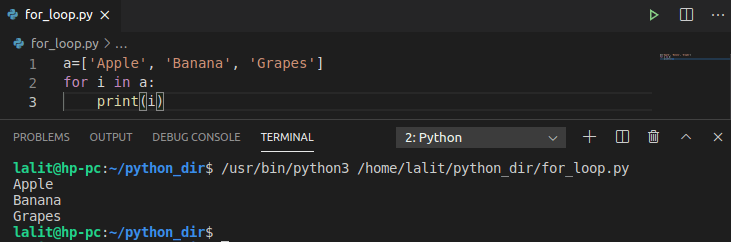# Python Loops

Report this app

## Description

A loop statement in programming is used to execute a line or block of code repeatedly. For example, suppose you want to print the “Hello World!” string 100 times you can do this in two ways either use a print statement 100 times or use a loop with a condition to run it 100 times. By using a loop you can do this in a few lines.

A loop in Python consists of two parts a condition and body, it executes until the given condition becomes false.

There are two types of loop used in Python –

• while loop
• far loop

In this article, we will discuss loops and their usage in Python with the help of examples.

Content Structure

## The while loop

The while loop is used to execute a line or block of code repeatedly until the condition is satisfied. Once the condition becomes false Python interpreter exits from the loop and executes the statement given after the loop statement.

The syntax of the while loop is given below –

```while condition:
statement(s)```

Now see the examples of using while loop in Python.

Example 1:

The following code will print “Hello World!” 10 times.

```i=0
while i<10:
print("Hello World!")
i+=1```

Ok, so now when you run this code this will print the “Hello World!” string 10 times you can see the output in the given image.Example 2:

The given lines of code will give you the sum of first 10 natural numbers i.e. sum of numbers from 1 to 10 now see how you can use while loop to find the sum.

```i=1
total=0
while i<=10:
total+=i
i+=1
print(f"The sum is {total}")```

You can see the output in the given image.## Python for loop

Python for loop serves the same purpose as while loop does. A for loop generally used for traversing string, array, list, etc. The range () function is used to iterate over a range.

The syntax of for loop in python is given below.

```for  iterator_var in range(start, end):
statement(s)```

or use the following to iterate over a sequence such as a string.

```for  iterator_var in sequnce:
statement(s)```

Now see the given Python for loop examples.

Example 1:

Here we will write code to print the “Hello World!” string 10 times using for loop.

```for i in range(0, 10):
print("Hello World!")```

Now you can see the output in the given image.Please note that the end value of the range function is excluded that means loop is running from 0 to 9 not 0 to 10.

Example 2:

You can use for loop to iterate over a collection of objects without specifying a condition or numeric value so it is also known as an iterator-based loop. For example –

```str="Hello World!"
for i in str:
print(i)```

You can see the output of this code in the given image –Example 3:

The following example also shows an iterator based loop, here we will iterate over the items of a list.

```a=['Apple', 'Banana', 'Grapes']
for i in a:
print(i)```

Now you can see the output in the given image –

##Nesting of loops

Python allows nesting of loops that means you can use a loop inside another loop. The syntax of the nesting of Python for and while loop is given below.

The syntax of nested while loop is –

```while condition:
while condition:
statement(s)
statement(s)```

The syntax of nested for loop is –

```for itrator_var in sequnce:
for iterator_var in sequnce:
statement(s)
statement(s)```

You can also use a for loop inside a while loop and a while loop inside a for loop.

Example of nested loops

```name = ["Sumit", "John", "Vinay"]
fruits = ["apple", "banana", "cherry"]
for i in name:
for j in fruits:
print(f"{i} eats {j}")```

When you execute this program you will see the following output.